• 问答
• 技术
• 实践
• 资源
TPAMI2020:无监督多类域适应：理论，算法与实践

1. 理论方面：提出了Multi-Class Scoring Disagreement (MCSD) divergence来衡量两个域数据分布之间的差异；其中MCSD可以充分衡量两个scoring hypotheses（可以理解为分类器） 之间的差异。基于MCSD divergence, 我们提出了新的Adaptation Bound， 并详细讨论了我们理论框架和之前框架的关系。
2. 算法方面：基于MCSD divergence 的理论，我们提出了一套新的代码框架Multi-class Domain-adversarial learning Networks (McDalNets)。McDalNets的不同实现与近期的流行方法相似或相同，因此从理论层面支撑了这些方法 [2,3,4,5]。此外，我们提出了一个新的算法SymmNets-V2, 该方法是我们之前会议工作的改进版本。
3. 实践方面：我们在closed set, partial, and open set 三种实验设置下验证了我们提出方法的有效性。代码链接：Code

## 理论方面：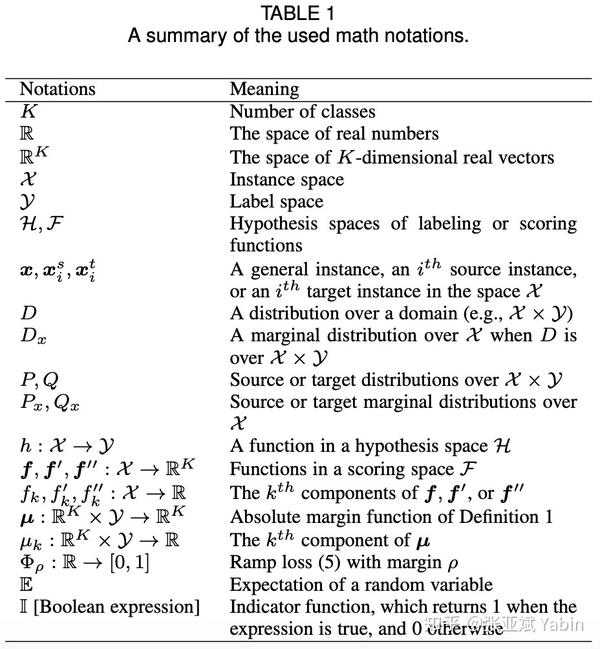$$d^{(\rho)}_{MCSD}(P_x, Qx) := \sup\limits{\boldsymbol{f'},\boldsymbol{f}''\in \mathcal{F}}[\text{MCSD}_{Qx}^{(\rho)}(\boldsymbol f', \boldsymbol f'') - \text{MCSD}{P_x}^{(\rho)}(\boldsymbol f', \boldsymbol f'')] ,$$

$$\boldsymbol M^{(\rho)}{i,j}(\boldsymbol f(\boldsymbol x)) = \Phi\rho(\mui(\boldsymbol f(\boldsymbol x),j)), \ \Phi\rho(x) := \begin{cases} 0, & \rho \leq x \ 1 - x/{\rho}, & 0 < x < \rho \ 1, & x \leq 0 \end{cases},\ \mu_k(\boldsymbol f(\boldsymbol x),y) = \begin{cases} +f_k(\boldsymbol x), & k = y \ -f_k(\boldsymbol x), & k \in \mathcal{Y} \setminus {y} \end{cases}.$$

$\Phi_\rho(x)$ 是ramp loss, $\muk(\boldsymbol f(\boldsymbol x),y)$ 指代absolute margin function $\boldsymbol u: \mathbb{R}^K \times \mathcal{Y} \rightarrow \mathbb{R}^K$ 的第 $k$ 个值。上述定义有些复杂，我们接下来对其直观描述： $\boldsymbol M^{(\rho)}$ 的每一列$\boldsymbol M^{(\rho)}{:,k}$ 计算了violations of absolute margin function $\boldsymbol u(\boldsymbol f(\cdot), k)$ ，进而 $| \boldsymbol M^{(\rho)}{:,k} (\boldsymbol f') -\boldsymbol M^{(\rho)}{:,k} (\boldsymbol f'')|_1$ 度量了 $\boldsymbol f', \boldsymbol f''$ 之间margin violations的差异，一个直观的例子如Fig 1(c)所示：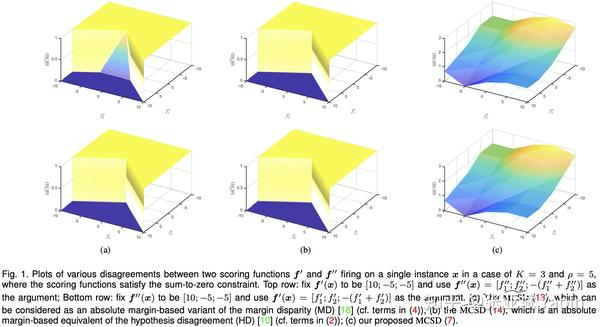$\widetilde{\text{MCSD}}D^{(\rho)}(\boldsymbol f', \boldsymbol f'') := \mathbb{E}{\boldsymbol x\sim D}\Phi{\rho/2}[\mu{h{\boldsymbol f''}(\boldsymbol x)}(\boldsymbol f''(\boldsymbol x),h{\boldsymbol f'}(\boldsymbol x))]$ 是absolute margin-based variant of margin disparity (MD) : $\mathbb{E}{ D}\Phi\rho(\rho{\boldsymbol f'}(\cdot, h{\boldsymbol f})) = \mathbb{E}{\boldsymbol x\sim D}\Phi\rho(\rho{\boldsymbol f'}(\boldsymbol x, h{\boldsymbol f}{(\boldsymbol x)}))$ , where $\rho_{\boldsymbol f}(\boldsymbol x, y) = \frac{1}{2} \left(fy(\boldsymbol x) - \max{y' \neq y} f_{y'}(\boldsymbol x) \right)$ 是relative margin function. 进而基于 $\widetilde{\text{MCSD}}_D^{(\rho)}(\boldsymbol f', \boldsymbol f'')$ 得到的divergence 是MDD 的变种。

$\widehat{\text{MCSD}}D^{(\rho)}(\boldsymbol f', \boldsymbol f'') := \mathbb{E}{\boldsymbol x\sim D}\mathbb{I}[\Phi{\rho}[\mu{h{\boldsymbol f''}(\boldsymbol x)}(\boldsymbol f''(\boldsymbol x),h{\boldsymbol f'}(\boldsymbol x))] = 1]$ 是ablolute margin-based equivalent of the hypothesis disagreement (HD) $\mathbb{E}_{Qx} \mathbb{I}[h\neq h'] = \mathbb{E}{\boldsymbol x\sim Q_x} \mathbb{I}[h(\boldsymbol x)\neq h'(\boldsymbol x)]$. 进而基于 $\widehat{\text{MCSD}}_D^{(\rho)}(\boldsymbol f', \boldsymbol f'')$ 得到的divergence 等效于 $\frac{1}{2}\mathcal{H}\Delta\mathcal{H}-divergence$

$\text{MCSD}_D^{(\rho)}(\boldsymbol f', \boldsymbol f'') ,\widetilde{\text{MCSD}}_D^{(\rho)}(\boldsymbol f', \boldsymbol f''), \widehat{\text{MCSD}}_D^{(\rho)}(\boldsymbol f', \boldsymbol f'')$ 作为3种不同的度量scoring functions差异的方法，其直观对比如Fig 1所示，可以总结如下：

• _$\widehat{\text{MCSD}}D^{(\rho)}(\boldsymbol f', \boldsymbol f'')$ 采用0-1二值loss只衡量了 $\boldsymbol f', \boldsymbol f''$ 的最终类别预测是否一致。
• _相对 $\widehat{\text{MCSD}}_D^{(\rho)}(\boldsymbol f', \boldsymbol f'')$ ，$\widetilde{\text{MCSD}}D^{(\rho)}(\boldsymbol f', \boldsymbol f'')$ 通过引入margin 在0和1之间做了一个平滑的过渡。
• _以上两者都只考虑了scoring functions的部分输出，$\text{MCSD}D^{(\rho)}(\boldsymbol f', \boldsymbol f'')$ 首次将scoring functions 的所有输出值加以考虑。故而MCSD可以充分度量scoring functions 的差异。

$\mathcal{E}Q(h{\boldsymbol f}) \leq \mathcal{E}^{(\rho)}P(\boldsymbol f) + d^{(\rho)}{MCSD}(P_x, Q_x) + \lambda$ ,其中 $\mathcal{E}Q(h{\boldsymbol f})$ 是targer error, $\mathcal{E}^{(\rho)}_P(\boldsymbol f)$ 是source error, $\lambda$ 可视为与数据集合hypothesis space相关的常数。相应的PAC bound也可以导出。

## 算法方面：

$$\min\limits{\boldsymbol f,\psi}\ \mathcal{E}^{(\rho)}{\widehat{P}^{\psi}}(\boldsymbol f) + [\text{MCSD}_{\widehat{Q}x^{\psi}}^{(\rho)}(\boldsymbol f', \boldsymbol f'') - \text{MCSD}{\widehat{P}x^{\psi}}^{(\rho)}(\boldsymbol f', \boldsymbol f'') ], \ \max\limits{\boldsymbol f',\boldsymbol f''}\ [\text{MCSD}_{\widehat{Q}x^{\psi}}^{(\rho)}(\boldsymbol f', \boldsymbol f'') - \text{MCSD}{\widehat{P}_x^{\psi}}^{(\rho)}(\boldsymbol f', \boldsymbol f'')] ,$$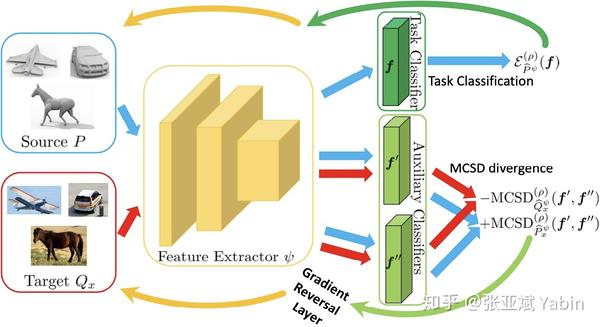1. 当 $\boldsymbol f', \boldsymbol f''$ 在domain $D$ 上的输出越趋于一致，替代度量方法的值越小
2. 当 $\boldsymbol f', \boldsymbol f''$ 在domain $D$ 上的输出越差异越大，替代度量方法的值越大

$$(L1/\text{MCD} ): \mathbb{E}{\boldsymbol x\sim D}\frac{1}{K}|\phi(\boldsymbol f'(\psi(\boldsymbol x)))-\phi(\boldsymbol f''(\psi(\boldsymbol x)))|_1$$

$$(\text{KL}): \mathbb{E}_{\boldsymbol x\sim D} \frac{1}{2}[\text{KL}(\phi(\boldsymbol f'(\psi(\boldsymbol x))), \phi(\boldsymbol f''(\psi(\boldsymbol x)))) + \text{KL}(\phi(\boldsymbol f''(\psi(\boldsymbol x))), \phi(\boldsymbol f'(\psi(\boldsymbol x))))]$$

$$(\text{CE}): \mathbb{E}_{\boldsymbol x\sim D} \frac{1}{2}[\text{CE}(\phi(\boldsymbol f'(\psi(\boldsymbol x))), \phi(\boldsymbol f''(\psi(\boldsymbol x)))) + \text{CE}(\phi(\boldsymbol f''(\psi(\boldsymbol x))), \phi(\boldsymbol f'(\psi(\boldsymbol x))))]$$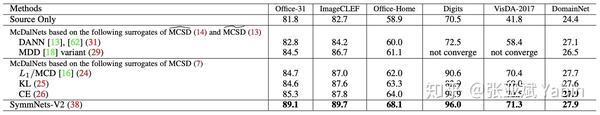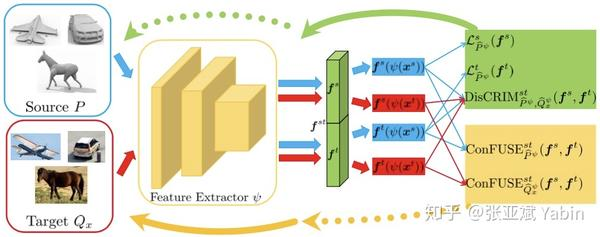$$\min{\psi} \text{ConFUSE}{\widehat{P}^{\psi}}^{st} (\boldsymbol f^{s},\boldsymbol f^t) + \lambda \text{ConFUSE}_{\widehat{Q}x^{\psi}}^{st} (\boldsymbol f^{s},\boldsymbol f^t), \ \min{\boldsymbol f^s, \boldsymbol f^t} {\cal{L}}{\widehat{P}^{\psi}}^s (\boldsymbol f^s) + {\cal{L}}{\widehat{P}^{\psi}}^t (\boldsymbol f^t) + \text{DisCRIM}_{\widehat{P}^{\psi}, \widehat{Q}_x^{\psi}}^{st} (\boldsymbol f^{s},\boldsymbol f^t)$$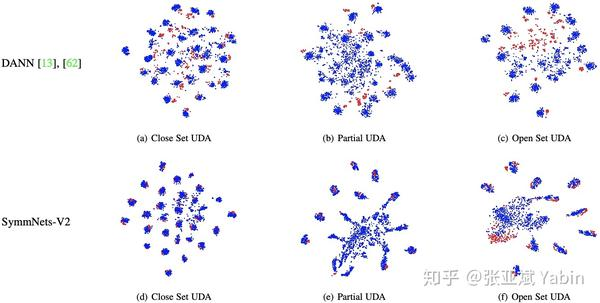Domain-Adversarial Training of Neural Networks, JMLR16
 Maximum Classifier Discrepancy for Unsupervised Domain Adaptation, CVPR18
 Domain-Symmetric Networks for Adversarial Domain Adaptation, CVPR19
 Unsupervised Domain Adaptation via Regularized Conditional Alignment, ICCV19
 Sliced wasserstein discrepancy for unsupervised domain adaptation, CVPR19
 Bridging Theory and Algorithm for Domain Adaptation, ICML19
 A theory of learning from different domains,ML10

•0
•0
•720收藏

• 0

关注
• 2

获赞
• 0

精选文章

• Cynthia-yawain的专栏

• 806
• 713
• 594
• 858
• 819